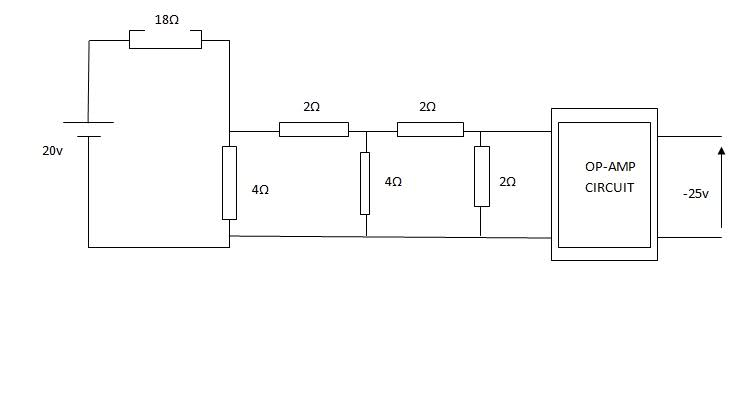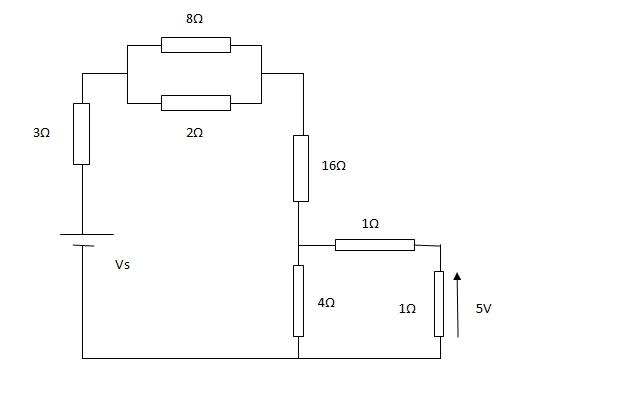# Simple Circuit Analysis - 2 Questions

Gorz
Appologies for the amazing circuit diagrams in MS Word

## Homework Statement

For the Circuit shown below, design the opamp circuit which will give an output of -25VFor the cicruit shown, calculate Vs## The Attempt at a Solution

Ok, for the first one, I summed the resistances in parallel and found that the the voltage 4 ohm resitor thats that would act as the voltage divider would have a value of 2 ohm, which means the volatge input using the voltage divider rule would be 2v, however the answers states that it is 0.5V

For the second question, , the 5V across the 1 ohm resistor, being in series with the other 1 ohm resistor, suggests that the sum of the voltages in that mini circuit is 10V. Resistors in parallel have the same voltage across, therefore, 10V across the 4ohm resistor. By the voltage divider rule, there should be 50V across the 16ohm resistor. Not sure how to go from here, ive tried playing around with figures for the other 2 in parallel and the 3ohm, and get nowhere near the answer of 164.48ohms

HELP!

Gorz
Sunday Afternoon Bump.

Staff Emeritus
Homework Helper

## The Attempt at a Solution

Ok, for the first one, I summed the resistances in parallel and found that the the voltage 4 ohm resitor thats that would act as the voltage divider would have a value of 2 ohm, which means the volatge input using the voltage divider rule would be 2v, however the answers states that it is 0.5V

Hmmm, I get 0.5V as well.

What equivalent resistance do you get when you combine all but the 18-ohm resistance? You can ignore the op-amp, since it behaves close to an open circuit as far as the resistor network is concerned.

Gorz
Hmmm, I get 0.5V as well.

What equivalent resistance do you get when you combine all but the 18-ohm resistance? You can ignore the op-amp, since it behaves close to an open circuit as far as the resistor network is concerned.

I get an equivalent resistance of 2ohms. Working from the Resistor closest to the Op-amp.

2+2 = 4 Ohms in series, 1/4 +1/4 = 0.5 = 2ohms.

2+2 = 4 Ohms in series, 1/4 +1/4 = 0.5 = 2ohms.

So the total resistance in the circuit is 20ohm. 20v source, therefore the input is 2v.

Where am I going wrong.

:(

Homework Helper
By the voltage divider rule, there should be 50V across the 16ohm resistor.
This is wrong. Find the current through 1 ohm and 4 ohm branch. That current flows through 16 ohm. I got that current 7.5 A. Now proceed.
So the total resistance in the circuit is 20ohm. 20v source, therefore the input is 2v
How did you get 2 V?
First of all find the current through each branch.

Last edited:
Gorz
By the voltage divider rule, there should be 50V across the 16ohm resistor.
This is wrong. Find the current through 1 ohm and 4 ohm branch. That current flows through 16 ohm. I got that current 7.5 A. Now proceed.

I assume you are talking about the 2nd question yes?

Homework Helper
Yes.

Staff Emeritus
Homework Helper
(1st question here)

I get an equivalent resistance of 2ohms. Working from the Resistor closest to the Op-amp.

2+2 = 4 Ohms in series, 1/4 +1/4 = 0.5 = 2ohms.

2+2 = 4 Ohms in series, 1/4 +1/4 = 0.5 = 2ohms.

So the total resistance in the circuit is 20ohm. 20v source, therefore the input is 2v.

Where am I going wrong.

:(

2 Ω (and 20 Ω if you add the 18 Ω resistor) is correct.

It's 2V across the network of 4Ω & 2Ω resistors, which means 2V across the left-most 4Ω resistor. But the voltage at the op-amp input (across the right-most 2Ω resistor) will be different.